### Output Data Sets

#### OUTEST= Data Set

The OUTEST= (or OUTVAR=) data set is of TYPE=EST and contains the final parameter estimates, the gradient, the Hessian, and boundary and linear constraints. For METHOD=ML, METHOD=GLS, and METHOD=WLS, the OUTEST= data set also contains the approximate standard errors, the information matrix (crossproduct Jacobian), and the approximate covariance matrix of the parameter estimates ((generalized) inverse of the information matrix). If there are linear or nonlinear equality or active inequality constraints at the solution, the OUTEST= data set also contains Lagrange multipliers, the projected Hessian matrix, and the Hessian matrix of the Lagrange function.

The OUTEST= data set can be used to save the results of an optimization by PROC CALIS for another analysis with either PROC CALIS or another SAS procedure. Saving results to an OUTEST= data set is advised for expensive applications that cannot be repeated without considerable effort.

The OUTEST= data set contains the BY variables, two character variables _TYPE_ and _NAME_, t numeric variables corresponding to the parameters used in the model, a numeric variable _RHS_ (right-hand side) that is used for the right-hand-side value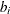of a linear constraint or for the value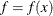of the objective function at the final point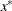of the parameter space, and a numeric variable _ITER_ that is set to zero for initial values, set to the iteration number for the OUTITER output, and set to missing for the result output.

The _TYPE_ observations in Table 27.5 are available in the OUTEST= data set, depending on the request.

Table 27.5: _TYPE_ Observations in the OUTEST= Data Set

_TYPE_

Description

ACTBC

If there are active boundary constraints at the solution, three observations indicate which of the parameters are actively constrained, as follows:

_NAME_

Description

GE

indicates the active lower bounds

LE

indicates the active upper bounds

EQ

COV

Contains the approximate covariance matrix of the parameter estimates; used in computing the approximate standard errors.

COVRANK

contains the rank of the covariance matrix of the parameter estimates.

CRPJ_LF

Contains the Hessian matrix of the Lagrange function (based on CRPJAC).

CRPJAC

Contains the approximate Hessian matrix used in the optimization process. This is the inverse of the information matrix.

EQ

If linear constraints are used, this observation contains the ith linear constraint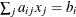. The parameter variables contain the coefficients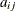,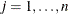, the _RHS_ variable contains, and _NAME_=ACTLC or _NAME_=LDACTLC.

GE

If linear constraints are used, this observation contains the ith linear constraint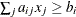. The parameter variables contain the coefficients,, and the _RHS_ variable contains. If the constraint i is active at the solution, then _NAME_=ACTLC or _NAME_=LDACTLC.

Contains the gradient of the estimates.

Contains the gradient of the Lagrange function. The _RHS_ variable contains the value of the Lagrange function.

HESSIAN

Contains the Hessian matrix.

HESS_LF

Contains the Hessian matrix of the Lagrange function (based on HESSIAN).

INFORMAT

Contains the information matrix of the parameter estimates (only for METHOD=ML, METHOD=GLS, or METHOD=WLS).

Contains the gradient of the starting estimates.

INITIAL

Contains the starting values of the parameter estimates.

JACNLC

Contains the Jacobian of the nonlinear constraints evaluated at the final estimates.

LAGM BC

Contains Lagrange multipliers for masks and active boundary constraints.

_NAME_

Description

GE

Indicates the active lower bounds

LE

Indicates the active upper bounds

EQ

LAGM LC

Contains Lagrange multipliers for linear equality and active inequality constraints in pairs of observations containing the constraint number and the value of the Lagrange multiplier.

_NAME_

Description

LEC_NUM

Number of the linear equality constraint

LEC_VAL

Corresponding Lagrange multiplier value

LIC_NUM

Number of the linear inequality constraint

LIC_VAL

Corresponding Lagrange multiplier value

LAGM NLC

contains Lagrange multipliers for nonlinear equality and active inequality constraints in pairs of observations that contain the constraint number and the value of the Lagrange multiplier.

_NAME_

Description

NLEC_NUM

Number of the nonlinear equality constraint

NLEC_VAL

Corresponding Lagrange multiplier value

NLIC_NUM

Number of the linear inequality constraint

NLIC_VAL

Corresponding Lagrange multiplier value

LE

If linear constraints are used, this observation contains the ith linear constraint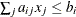. The parameter variables contain the coefficients,, and the _RHS_ variable contains. If the constraint i is active at the solution, then _NAME_=ACTLC or _NAME_=LDACTLC.

LOWERBD
| LB

If boundary constraints are used, this observation contains the lower bounds. Those parameters not subjected to lower bounds contain missing values. The _RHS_ variable contains a missing value, and the _NAME_ variable is blank.

NACTBC

All parameter variables contain the number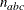of active boundary constraints at the solution. The _RHS_ variable contains a missing value, and the _NAME_ variable is blank.

NACTLC

All parameter variables contain the number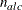of active linear constraints at the solutionthat are recognized as linearly independent. The _RHS_ variable contains a missing value, and the _NAME_ variable is blank.

NLC_EQ
NLC_GE
NLC_LE

Contains values and residuals of nonlinear constraints. The _NAME_ variable is described as follows:

_NAME_

Description

NLC

Inactive nonlinear constraint

NLCACT

Linear independent active nonlinear constraint

NLCACTLD

Linear dependent active nonlinear constraint

NLDACTBC

Contains the number of active boundary constraints at the solutionthat are recognized as linearly dependent. The _RHS_ variable contains a missing value, and the _NAME_ variable is blank.

NLDACTLC

Contains the number of active linear constraints at the solutionthat are recognized as linearly dependent. The _RHS_ variable contains a missing value, and the _NAME_ variable is blank.

_NOBS_

Contains the number of observations.

PARMS

Contains the final parameter estimates. The _RHS_ variable contains the value of the objective function.

PCRPJ_LF

Contains the projected Hessian matrix of the Lagrange function (based on CRPJAC).

PHESS_LF

Contains the projected Hessian matrix of the Lagrange function (based on HESSIAN).

PROJCRPJ

Contains the projected Hessian matrix (based on CRPJAC).

If linear constraints are used in the estimation, this observation contains the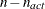values of the projected gradient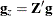in the variables corresponding to the firstparameters. The _RHS_ variable contains a missing value, and the _NAME_ variable is blank.

PROJHESS

Contains the projected Hessian matrix (based on HESSIAN).

STDERR

Contains approximate standard errors (only for METHOD=ML, METHOD=GLS, or METHOD=WLS).

TERMINAT

The _NAME_ variable contains the name of the termination criterion.

UPPERBD
| UB

If boundary constraints are used, this observation contains the upper bounds. Those parameters not subjected to upper bounds contain missing values. The _RHS_ variable contains a missing value, and the _NAME_ variable is blank.

If the technique specified by the OMETHOD= option cannot be performed (for example, no feasible initial values can be computed or the function value or derivatives cannot be evaluated at the starting point), the OUTEST= data set can contain only some of the observations (usually only the PARMS and GRAD observations).

#### OUTMODEL= or OUTRAM= Data Set

The OUTMODEL= (or OUTRAM=) data set is of TYPE=CALISMDL and contains the model specification, the computed parameter estimates, and the standard error estimates. This data set is intended to be reused as an INMODEL= data set to specify good initial values in a subsequent analysis by PROC CALIS.

The OUTMODEL= data set contains the following variables:

• the BY variables, if any

• an _MDLNUM_ variable for model numbers, if used

• a character variable _TYPE_, which takes various values that indicate the type of model specification

• a character variable _NAME_, which indicates the model type, parameter name, or variable name

• a character variable _MATNR_, which indicates the matrix number (COSAN models only)

• a character variable _VAR1_, which is the name or number of the first variable in the specification

• a character variable _VAR2_, which is the name or number of the second variable in the specification

• a numerical variable _ESTIM_ for the final estimate of the parameter location

• a numerical variable _STDERR_ for the standard error estimate of the parameter location

• a numerical variable _SDEST_ for the final standardized estimate of the parameter location

• a numerical variable _SDSE_ for the standard error of the standardized estimate of the parameter location

Although the _SDEST_ and _SDSE_ variables are created for COSAN models, the values for these two variables are always missing because there are no rules to carry out the standardization of COSAN models.

Each observation (record) of the OUTMODEL= data set contains a piece of information regarding the model specification. Depending on the type of the specification indicated by the value of the _TYPE_ variable, the meanings of _NAME_, _VAR1_, and _VAR2_ differ. The following tables summarize the meanings of the _NAME_, _MATNR_ (COSAN models only), _VAR1_, and _VAR2_ variables for each value of the _TYPE_ variable, given the type of the model.

##### COSAN Models

_TYPE_=

Description

_NAME_

_MATNR_

_VAR1_

_VAR2_

MDLTYPE

Model type

COSAN

VAR

Variable

Variable name

Matrix number

Column location

MATRIX

Matrix

Matrix name

Matrix number

Number of rows

Number of columns

MODEL

Model formula

COV or MEAN

Matrix number

Term number

Location in term

ESTIM

Parameters

Parameter name

Matrix number

Row number

Column number

The value of the _NAME_ variable is COSAN for the _TYPE_=MDLTYPE observation.

The _TYPE_=VAR observations store the information about the column variables in matrices. The _NAME_ variable stores the variable names. The value of _VAR1_ indicates the column location of the variable in the matrix with the matrix number stored in _MATNR_.

The _TYPE_=MATRIX observations store the information about the model matrices. The _NAME_ variable stores the matrix names. The value of _MATNR_ indicates the corresponding matrix number. The values of_VAR1_ and _VAR2_ indicates the numbers of rows and columns, respectively, of the matrix.

The _TYPE_=MODEL observations store the covariance and mean structure formulas. The _NAME_ variable indicates whether the mean (MEAN) or covariance (COV) structure information is stored. The value of _MATNR_ indicates the matrix number in the mean or covariance structure formula. The _VAR1_ variable indicates the term number, and the _VAR2_ variable indicates the location of the matrix in the term.

The _TYPE_=ESTIM observations store the information about the parameters and their estimates. The _NAME_ variable stores the parameter names. The value of _MATNR_ indicates the matrix number. The values of _VAR1_ and _VAR2_ indicate the associated row and column numbers, respectively, of the parameter.

##### FACTOR Models

_TYPE_=

Description

_NAME_

_VAR1_

_VAR2_

MDLTYPE

Model type

Model type

FACTVAR

Variable

Variable name

Variable number

Variable type

Parameter name

Manifest variable

Factor variable

COV

Covariance

Parameter name

First variable

Second variable

PVAR

(Partial) variance

Parameter name

Variable

MEAN

Mean or intercept

Parameter name

Variable

Parameter name

First variable

Second variable

Parameter name

Variable

Parameter name

Variable

For factor models, the value of the _NAME_ variable is either EFACTOR (exploratory factor model) or CFACTOR (confirmatory factor model) for the _TYPE_=MDLTYPE observation.

The _TYPE_=FACTVAR observations store the information about the variables in the model. The _NAME_ variable stores the variable names. The value of _VAR1_ indicates the variable number. The value of _VAR2_ indicates the type of the variable: either DEPV for dependent observed variables or INDF for latent factors.

Other observations specify the parameters and their estimates in the model. The _NAME_ values for these observations are the parameter names. Observation with _TYPE_=LOADING, _TYPE_=COV, or _TYPE_=ADDCOV are for parameters that are associated with two variables. The _VAR1_ and _VAR2_ values of these two types of observations indicate the variables involved.

Observations with _TYPE_=PVAR, _TYPE_=MEAN, _TYPE_=ADDPVAR, or _TYPE_=ADDMEAN are for parameters that are associated with a single variable. The value of _VAR1_ indicates the variable involved.

##### LINEQS Models

_TYPE_=

Description

_NAME_

_VAR1_

_VAR2_

MDLTYPE

Model type

LINEQS

EQSVAR

Variable

Variable name

Variable number

Variable type

EQUATION

Path coefficient

Parameter

Outcome variable

Predictor variable

COV

Covariance

Parameter

First variable

Second variable

VARIANCE

Variance

Parameter

Variable

MEAN

Mean

Parameter

Variable

Parameter

First variable

Second variable

Parameter

Variable

Parameter

Variable

Parameter

Variable

The value of the _NAME_ variable is LINEQS for the _TYPE_=MDLTYPE observation.

The _TYPE_=EQSVAR observations store the information about the variables in the model. The _NAME_ variable stores the variable names. The value of _VAR1_ indicates the variable number. The value of _VAR2_ indicates the type of the variable. There are six types of variables in the LINEQS model:

• DEPV for dependent observed variables

• INDV for independent observed variables

• DEPF for dependent latent factors

• INDF for independent latent factors

• INDD for independent error terms

• INDE for independent disturbance terms

Other observations specify the parameters and their estimates in the model. The _NAME_ values for these observations are the parameter names. Observation with _TYPE_=EQUATION, _TYPE_=COV, or _TYPE_=ADDCOV are for parameters that are associated with two variables. The _VAR1_ and _VAR2_ values of these two types of observations indicate the variables involved.

Observations with _TYPE_=VARIANCE, _TYPE_=MEAN, _TYPE_=ADDVARIA, _TYPE_=ADDINTE, or _TYPE_=ADDMEAN are for parameters associated with a single variable. The value of _VAR1_ indicates the variable involved.

##### LISMOD Models

_TYPE_=

Description

_NAME_

_VAR1_

_VAR2_

MDLTYPE

model type

LISMOD

XVAR-variable

Variable

Variable number

YVAR-variable

Variable

Variable number

ETAVAR
-variable

Variable

Variable number

XIVAR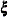-variable

Variable

Variable number

ALPHA

_ALPHA_ entry

Parameter

Row number

BETA

_BETA_ entry

Parameter

Row number

Column number

GAMMA

_BETA_ entry

Parameter

Row number

Column number

KAPPA

_KAPPA_ entry

Parameter

Row number

LAMBDAX

_LAMBDAX_ entry

Parameter

Row number

Column number

LAMBDAY

_LAMBDAY_ entry

Parameter

Row number

Column number

NUX

_NUX_ entry

Parameter

Row number

NUY

_NUY_ entry

Parameter

Row number

PHI

_PHI_ entry

Parameter

Row number

Column number

PSI

_PSI_ entry

Parameter

Row number

Column number

THETAX

_THETAX_ entry

Parameter

Row number

Column number

THETAY

_THETAY_ entry

Parameter

Row number

Column number

Parameter

Row number

Parameter

Row number

Parameter

Row number

Parameter

Row number

Parameter

Row number

Column number

Parameter

Row number

Column number

Parameter

Row number

Column number

Parameter

Row number

Column number

The value of the _NAME_ variable is LISMOD for the _TYPE_=MDLTYPE observation. Other observations specify either the variables or the parameters in the model.

Observations with _TYPE_ values equal to XVAR, YVAR, ETAVAR, and XIVAR indicate the variables in the respective lists in the model. The _NAME_ variable of these observations stores the names of the variables, and the _VAR1_ variable stores the variable numbers in the respective list. The variable numbers in this data set are not arbitrary—that is, they define the variable orders in the rows and columns of the LISMOD model matrices. The _VAR2_ variable of these observations is not used.

All other observations in this data set specify the parameters in the model. The _NAME_ values of these observations are the parameter names. The corresponding _VAR1_ and _VAR2_ values of these observations indicate the row and column locations of the parameters in the LISMOD model matrices that are specified in the _TYPE_ variable. For example, when the value of _TYPE_ is ADDPHI or PHI, the parameter specified is located in the _PHI_ matrix, with its row and column numbers indicated by the _VAR1_ and _VAR2_ values, respectively. Some observations for specifying parameters do not have values in the _VAR2_ variable. This means that the associated LISMOD matrices are vectors so that the column numbers are always 1 for these observations.

##### MSTRUCT Models

_TYPE_=

Description

_NAME_

_VAR1_

_VAR2_

MDLTYPE

Model type

MSTRUCT

VAR

Variable

Variable

Variable number

COVMAT

Covariance

Parameter

Row number

Column number

MEANVEC

Mean

Parameter

Row number

Parameter

Row number

Column number

AMEANVEC

Parameter

Row number

The value of the _NAME_ variable is MSTRUCT for the _TYPE_=MDLTYPE observation. Other observations specify either the variables or the parameters in the model.

Observations with _TYPE_ values equal to VAR indicate the variables in the model. The _NAME_ variable of these observations stores the names of the variables, and the _VAR1_ variable stores the variable numbers in the variable list. The variable numbers in this data set are not arbitrary—that is, they define the variable orders in the rows and columns of the mean and covariance matrices. The _VAR2_ variable of these observations is not used.

All other observations in this data set specify the parameters in the model. The _NAME_ values of these observations are the parameter names. The corresponding _VAR1_ and _VAR2_ values of these observations indicate the row and column locations of the parameters in the mean or covariance matrix, as specified in the _TYPE_ model. For example, when _TYPE_=COVMAT, the parameter specified is located in the covariance matrix, with its row and column numbers indicated by the _VAR1_ and _VAR2_ values, respectively. For observations with _TYPE_=MEANVEC, the _VAR2_ variable is not used because the column numbers are always 1 for parameters in the mean vector.

##### PATH Models

_TYPE_=

Description

_NAME_

_VAR1_

_VAR2_

MDLTYPE

Model type

PATH

PATHVAR

Variable

Variable name

Variable number

Variable type

LEFT

Path coefficient

Parameter

Outcome variable

Predictor variable

RIGHT

Path coefficient

Parameter

Predictor variable

Outcome variable

PCOV

(Partial) covariance

Parameter

First variable

Second variable

PCOVPATH

(Partial) covariance path

Parameter

First variable

Second variable

PVAR

(Partial) variance

Parameter

Variable

PVARPATH

(Partial) variance path

Parameter

Variable

Variable

MEAN

Mean or intercept

Parameter

Variable

ONEPATH

Mean or intercept path

Parameter

_ONE_

Variable

Parameter

First variable

Second variable

Parameter

Variable

Parameter

Variable

The value of the _NAME_ variable is PATH for the _TYPE_=MDLTYPE observation.

The _TYPE_=PATHVAR observations store the information about the variables in the model. The _NAME_ variable stores the variable names. The value of _VAR1_ indicates the variable number. The value of _VAR2_ indicates the type of the variable. There are four types of variables in the PATH model:

• DEPV for dependent observed variables

• INDV for independent observed variables

• DEPF for dependent latent factors

• INDF for independent latent factors

Other observations specify the parameters in the model. The _NAME_ values for these observations are the parameter names. Observation with _TYPE_=LEFT, _TYPE_=RIGHT, _TYPE_=PCOV, or _TYPE_=ADDPCOV are for parameters that are associated with two variables. The _VAR1_ and _VAR2_ values of these two types of observations indicate the variables involved.

Observations with _TYPE_=PVAR, _TYPE_=MEAN, _TYPE_=ADDPVAR, or _TYPE_=ADDMEAN are for parameters that are associated with a single variable. The value of _VAR1_ indicates the variable involved.

##### RAM Models

_TYPE_=

Description

_NAME_

_VAR1_

_VAR2_

MDLTYPE

Model type

RAM

RAMVAR

Variable name

Variable

Variable number

Variable type

_A_

_A_ entry

Parameter

Row number

Column number

_P_

_P_ entry

Parameter

Row number

Column number

_W_

_W_ entry

Parameter

Row number

Column number

Added _P_ entry

Parameter

Row number

Column number

Added _W_ entry

Parameter

Row number

Column number

The value of the _NAME_ variable is RAM for the _TYPE_=MDLTYPE observation.

For the _TYPE_=RAMVAR observations, the _NAME_ variable stores the variable names, the _VAR1_ variable stores the variable number, and the _VAR2_ variable stores the variable type. There are four types of variables in the PATH model:

• DEPV for dependent observed variables

• INDV for independent observed variables

• DEPF for dependent latent factors

• INDF for independent latent factors

Other observations specify the parameters in the model. The _NAME_ variable stores the parameter name. The _TYPE_ variable indicates the associated matrix with the row number indicated in _VAR1_ and column number indicated in _VAR2_.

##### Reading an OUTMODEL= Data Set As an INMODEL= Data Set in Subsequent Analyses

When the OUTMODEL= data set is treated as an INMODEL= data set in subsequent analyses, you need to pay attention to observations with _TYPE_ values prefixed by ADD, AD, or A (for example, ADDCOV, ADTHETAY, or AMEANVEC). These observations represent default parameter locations that are generated by PROC CALIS in a previous run. Because the context of the new analyses might be different, these observations for added parameter locations might no longer be suitable in the new runs. Hence, these observations are not read as input model information. Fortunately, after reading the INMODEL= specification in the new analyses, CALIS analyzes the new model specification again. It then adds an appropriate set of parameters in the new context when necessary. If you are certain that the added parameter locations in the INMODEL= data set are applicable, you can force the input of these observations by using the READADDPARM option in the PROC CALIS statement. However, you must be very careful about using the READADDPARM option. The added parameters from the INMODEL= data set might have the same parameter names as those for the generated parameters in the new run. This might lead to unnecessary constraints in the model.

#### OUTSTAT= Data Set

The OUTSTAT= data set is similar to the TYPE=COV, TYPE=UCOV, TYPE=CORR, or TYPE=UCORR data set produced by the CORR procedure. The OUTSTAT= data set contains the following variables:

• the BY variables, if any

• the _GPNUM_ variable for groups numbers, if used in the analysis

• two character variables, _TYPE_ and _NAME_

• the manifest and the latent variables analyzed

The OUTSTAT= data set contains the following information (when available) in the observations:

• the mean and standard deviation

• the skewness and kurtosis (if the DATA= data set is a raw data set and the KURTOSIS option is specified)

• the number of observations

• if the WEIGHT statement is used, sum of the weights

• the correlation or covariance matrix to be analyzed

• the robust covariances, standard deviations, and means for robust estimation

• the predicted correlation or covariance matrix

• the standardized or normalized residual correlation or covariance matrix

• if the model contains latent variables, the predicted covariances between latent and manifest variables and the latent variable (or factor) score regression coefficients (see the )

In addition, for FACTOR models the OUTSTAT= data set contains:

• the unrotated factor loadings, the error variances, and the matrix of factor correlations

If effects are analyzed, the OUTSTAT= data set also contains:

• direct, indirect, and total effects and their standard error estimates

• standardized direct, indirect, and total effects and their standard error estimates

Each observation in the OUTSTAT= data set contains some type of statistic as indicated by the _TYPE_ variable. The values of the _TYPE_ variable are shown in the following tables:

##### Basic Descriptive Statistics

Value of _TYPE_

Contents

CORR

Correlations

COV

Covariances

KURTOSIS

Univariate kurtosis

MEAN

Means

N

Sample size

NPARTIAL

Number of partial variables

PARTCOV

Covariances after partialling

PARTCORR

Correlations after partialling

PARTMEAN

Means after partialling

PARTSTD

Standard deviations after partialling

ROBCOV

Robust covariances

ROBMEAN

Robust means

ROBSTD

Robust standard deviations

SKEWNESS

Univariate skewness

STD

Standard deviations

SUMWGT

Sum of weights (if the WEIGHT statement is used)

VARDIV

Variance divisor

VARDIVAJ

For the _TYPE_=CORR or COV observations, the _NAME_ variable contains the name of the manifest variable that corresponds to each row for the covariance or correlation. For other observations, _NAME_ is blank.

##### Predicted Moments and Residuals

value of _TYPE_

Contents

METHOD=DWLS

DWLSPRED

DWLS predicted moments

DWLSRES

DWLS raw residuals

DWLSSRES

DWLS variance standardized residuals

METHOD=GLS

GLSASRES

GLS asymptotically standardized residuals

GLSNRES

GLS normalized residuals

GLSPRED

GLS predicted moments

GLSRES

GLS raw residuals

GLSSRES

GLS variance standardized residuals

METHOD=ML or FIML

MAXASRES

ML asymptotically standardized residuals

MAXNRES

ML normalized residuals

MAXPRED

ML predicted moments

MAXRES

ML raw residuals

MAXSRES

ML variance standardized residuals

METHOD=ULS

ULSPRED

ULS predicted moments

ULSRES

ULS raw residuals

ULSSRES

ULS variance standardized residuals

METHOD=WLS

WLSASRES

WLS asymptotically standardized residuals

WLSNRES

WLS normalized residuals

WLSPRED

WLS predicted moments

WLSRES

WLS raw residuals

WLSSRES

WLS variance standardized residuals

For residuals or predicted moments of means, the _NAME_ variable is a fixed value denoted by _Mean_. For residuals or predicted moments for covariances or correlations, the _NAME_ variable is used for names of variables.

##### Effects and Latent Variable Scores Regression Coefficients

Value of _TYPE_

Contents

Unstandardized Effects

DEFFECT

Direct effects

DEFF_SE

Standard error estimates for direct effects

IEFFECT

Indirect effects

IEFF_SE

Standard error estimates for indirect effects

TEFFECT

Total effects

TEFF_SE

Standard error estimates for total effects

Standardized Effects

SDEFF

Standardized direct effects

SDEFF_SE

Standard error estimates for standardized direct effects

SIEFF

Standardized indirect effects

SIEFF_SE

Standard error estimates for standardized indirect effects

STEFF

Standardized total effects

STEFF_SE

Standard error estimates for standardized total effects

Latent Variable Scores Coefficients

LSSCORE

Latent variable (or factor) scores regression coefficients for ULS method

SCORE

Latent variable (or factor) scores regression coefficients other than ULS method

For latent variable or factor scores coefficients, the _NAME_ variable contains factor or latent variables in the observations. For other observations, the _NAME_ variable contains manifest or latent variable names.

You can use the latent variable score regression coefficients with PROC SCORE to compute factor scores. If the analyzed matrix is a covariance rather than a correlation matrix, the _TYPE_=STD observation is not included in the OUTSTAT= data set. In this case, the standard deviations can be obtained from the diagonal elements of the covariance matrix. Dropping the _TYPE_=STD observation prevents PROC SCORE from standardizing the observations before computing the factor scores.

##### Factor Analysis Results

Value of _TYPE_

Contents

ERRVAR

Error variances

FCOV

Factor correlations or covariances

RFCOV

Rotated factor correlations or covariances

ROTMAT

Rotation matrix

STDFCOV

Standardized factor correlations

STDRFCOV

Standardized rotated factor correlations or covariances

For the _TYPE_=ERRVAR observation, the _NAME_ variable is blank. For all other observations, the _NAME_ variable contains factor names.

#### OUTWGT= Data Set

You can create an OUTWGT= data set that is of TYPE=WEIGHT and contains the weight matrix used in generalized, weighted, or diagonally weighted least squares estimation. The OUTWGT= data set contains the weight matrix on which the WRIDGE= and the WPENALTY= options are applied. However, if you input the inverse of the weight matrix with the INWGT= and INWGTINV options (or the INWGT(INV)= option alone) in the same analysis, the OUTWGT= data set contains the same elements of the inverse of the weight matrix. For unweighted least squares or maximum likelihood estimation, no OUTWGT= data set can be written. The weight matrix used in maximum likelihood estimation is dynamically updated during optimization. When the ML solution converges, the final weight matrix is the same as the predicted covariance or correlation matrix, which is included in the OUTSTAT= data set (observations with _TYPE_ =MAXPRED).

For generalized and diagonally weighted least squares estimation, the weight matrices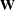of the OUTWGT= data set contain all elements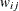, where the indices i and j correspond to all manifest variables used in the analysis. Let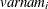be the name of the ith variable in the analysis. In this case, the OUTWGT= data set contains n observations with the variables shown in the following table:

Variable

Contents

_TYPE_

WEIGHT (character)

_NAME_

Name of variable(character)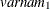Weight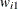for variable(numeric)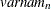Weight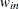for variable(numeric)

For weighted least squares estimation, the weight matrixof the OUTWGT= data set contains only the nonredundant elements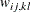. In this case, the OUTWGT= data set contains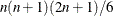observations with the variables shown in the following table:

Variable

Contents

_TYPE_

WEIGHT (character)

_NAME_

Name of variable(character)

_NAM2_

Name of variable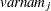(character)

_NAM3_

Name of variable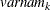(character)Weight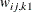for variable(numeric)Weight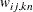for variable(numeric)

Symmetric redundant elements are set to missing values.

#### OUTFIT= Data Set

You can create an OUTFIT= data set that is of TYPE=CALISFIT and that contains the values of the fit indices of your analysis. If you use two estimation methods such as LSML or LSWLS, the fit indices are for the second analysis. An OUTFIT=data set contains the following variables:

• a character variable _TYPE_ for the types of fit indices

• a numerical variable IndexCode for the codes of the fit indices

• a character variable FitIndex for the names of the fit indices

• a numerical variable FitValue for the numerical values of the fit indices

• a character variable PrintChar for the character-formatted fit index values.

The possible values of _TYPE_ are:

ModelInfo:

basic modeling statistics and information

Absolute:

stand-alone fit indices

Parsimony:

fit indices that take model parsimony into account

Incremental:

fit indices that are based on comparison with a baseline model

##### Possible Values of FitIndex When _TYPE_=ModelInfo

Value of FitIndex

Description

Number of Observations

Number of observations used in the analysis

Number of Complete Observations

Number of complete observations (METHOD=FIML)

Number of Incomplete Observations

Number of incomplete observations (METHOD=FIML)

Number of Variables

Number of variables

Number of Moments

Number of mean or covariance elements

Number of Parameters

Number of parameters

Number of Active Constraints

Number of active constraints in the solution

Saturated Model Estimation

Estimation status of the saturated model (METHOD=FIML)

Saturated Model Function Value

Saturated model function value (METHOD=FIML)

Saturated Model -2 Log-Likelihood

Saturated model –2 log-likelihood function value (METHOD=FIML)

Baseline Model Estimation

Estimation status of the baseline model (METHOD=FIML)

Baseline Model Function Value

Baseline model function value

Baseline Model -2 Log-Likelihood

Baseline model –2 log-likelihood function value (METHOD=FIML)

Baseline Model Chi-Square

Baseline model chi-square value

Baseline Model Chi-Square DF

Baseline model chi-square degrees of freedom

Baseline Model DF

Baseline model degrees of freedom (METHOD=ULS or METHOD=DWLS)

Pr > Baseline Model Chi-Square

p value of the baseline model chi-square

##### Possible Values of FitIndex When _TYPE_=Absolute

Value of FitIndex

Description

Fit Function

Fit function value

-2 Log-Likelihood

–2 log-likelihood function value for the model (METHOD=FIML)

Chi-Square

Model chi-square value

Chi-Square DF

Degrees of freedom for the model chi-square test

Model DF

Degrees of freedom for model (METHOD=ULS or METHOD=DWLS)

Pr > Chi-Square

Probability of obtaining a larger chi-square than the observed value

Percent Contribution to Chi-Square

Percentage contribution to the chi-square value

Percent Contribution to Likelihood

Percentage contribution to the –2 log-likelihood function value (METHOD=FIML)

Elliptic Corrected Chi-Square

Elliptic-corrected chi-square value

Pr > Elliptic Corr. Chi-Square

Probability of obtaining a larger elliptic-corrected chi-square value

Z-test of Wilson and Hilferty

Z-test of Wilson and Hilferty

Hoelter Critical N

N value that makes a significant chi-square when multiplied to the fit function value

Root Mean Square Residual (RMR)

Root mean square residual

Standardized RMR (SRMR)

Standardized root mean square residual

Goodness of Fit Index (GFI)

Jöreskog and Sörbom goodness-of-fit index

##### Possible Values of FitIndex When _TYPE_=Parsimony

Value of FitIndex

Description

Goodness-of-fit index adjusted for the degrees of freedom of the model

Parsimonious GFI

Mulaik et al. (1989) modification of the GFI

RMSEA Estimate

Steiger and Lind (1980) root mean square error approximation

RMSEA Lower r% Confidence Limit

Lower r%confidence limit for RMSEA

RMSEA Upper r% Confidence Limit

Upper r%confidence limit for RMSEA

Probability of Close Fit

Browne and Cudeck (1993) test of close fit

ECVI Estimate

Expected cross-validation index

ECVI Lower r% Confidence Limit

Lower r%confidence limit for ECVI

ECVI Upper r% Confidence Limit

Upper r%confidence limit for ECVI

Akaike Information Criterion

Akaike information criterion

Bozdogan CAIC

Bozdogan (1987) consistent AIC

Schwarz Bayesian Criterion

Schwarz (1978) Bayesian criterion

McDonald Centrality

McDonald and Marsh (1988) measure of centrality

1. The value of r is one minus the ALPHARMS= value. By default, r=90. 2. The value of r is one minus the ALPHAECV= value. By default, r=90.

##### Possible Values of FitIndex When _TYPE_=Incremental

Value of FitIndex

Description

Bentler Comparative Fit Index

Bentler (1985) comparative fit index

Bentler-Bonett NFI

Bentler and Bonett (1980) normed fit index

Bentler-Bonett Non-normed Index

Bentler and Bonett (1980) nonnormed fit index

Bollen Normed Index Rho1

Bollen normed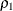Bollen Non-normed Index Delta2

Bollen nonnormed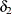James et al. Parsimonious NFI

James, Mulaik, and Brett (1982) parsimonious normed fit index